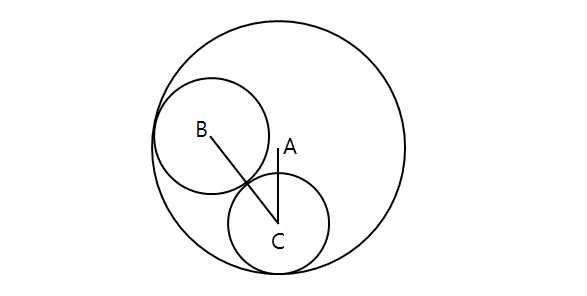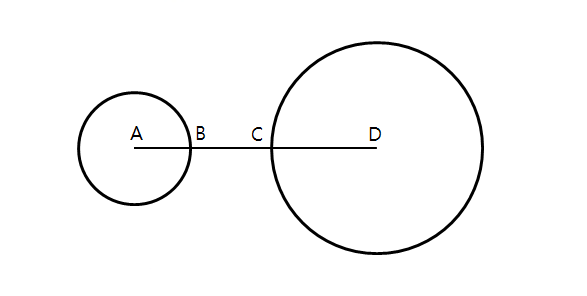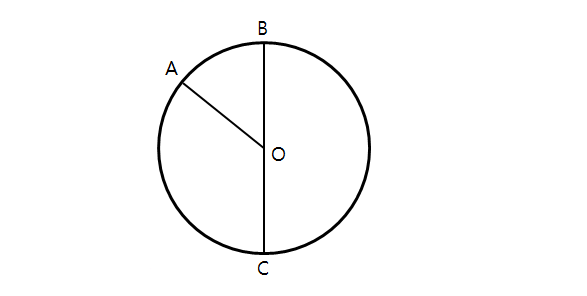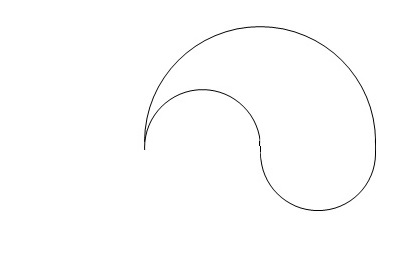Geometry

# Circles - Radius and Diameter and CircumferenceIn the above diagram, circles $C$ and $B$ are both externally tangent to each other and inscribed in circle $A.$ The radius of circle $A$ is $11,$ the radius of circle $B$ is $6,$ and the radius of circle $C$ is $5.$ What is the sum of the lengths of $\overline{AC}$ and $\overline{BC}?$

Note: The above diagram is not drawn to scale.Consider two circles with radius $5$ and $14,$ respectively. If $d$ denotes the distance between the circle centers, and the number of lines that are tangent to both circles is $4,$ what is the range of $d$?Consider two fixed circles with different radii as shown in the above diagram. If the distance between their centers, denoted $\lvert \overline{AD} \rvert,$ is $12,$ then the two circles become externally tangent to each other. If $\lvert \overline{AD} \rvert=4,$ the smaller circle becomes inscribed in the larger one. What is the radius of the larger circle?In the above diagram, $O$ is the center of the circle and the length of $\overline{OA}$ is $4.$ What is the length of $\overline{BC}?$The above partial yin yang symbol is created from a large semicircle with radius $14$ by removing one semicircle with radius $7$ on the left and adding one semicircle with radius $7$ on the right. What is the circumference of the symbol?

×